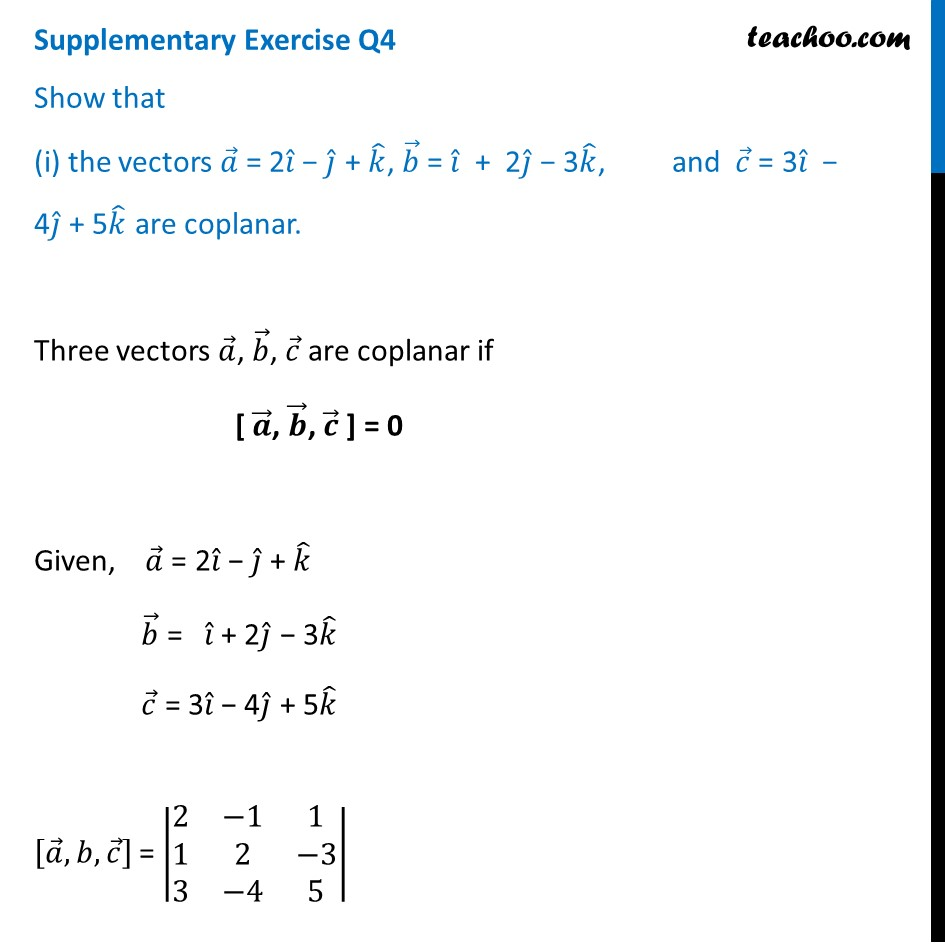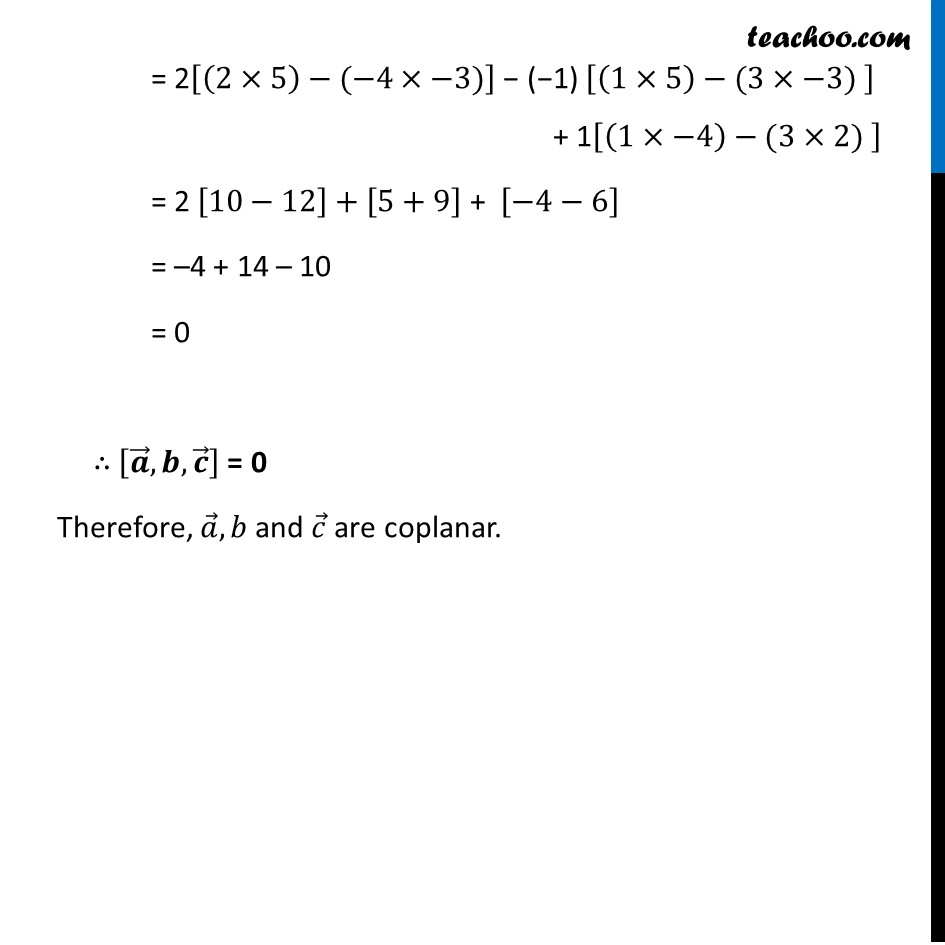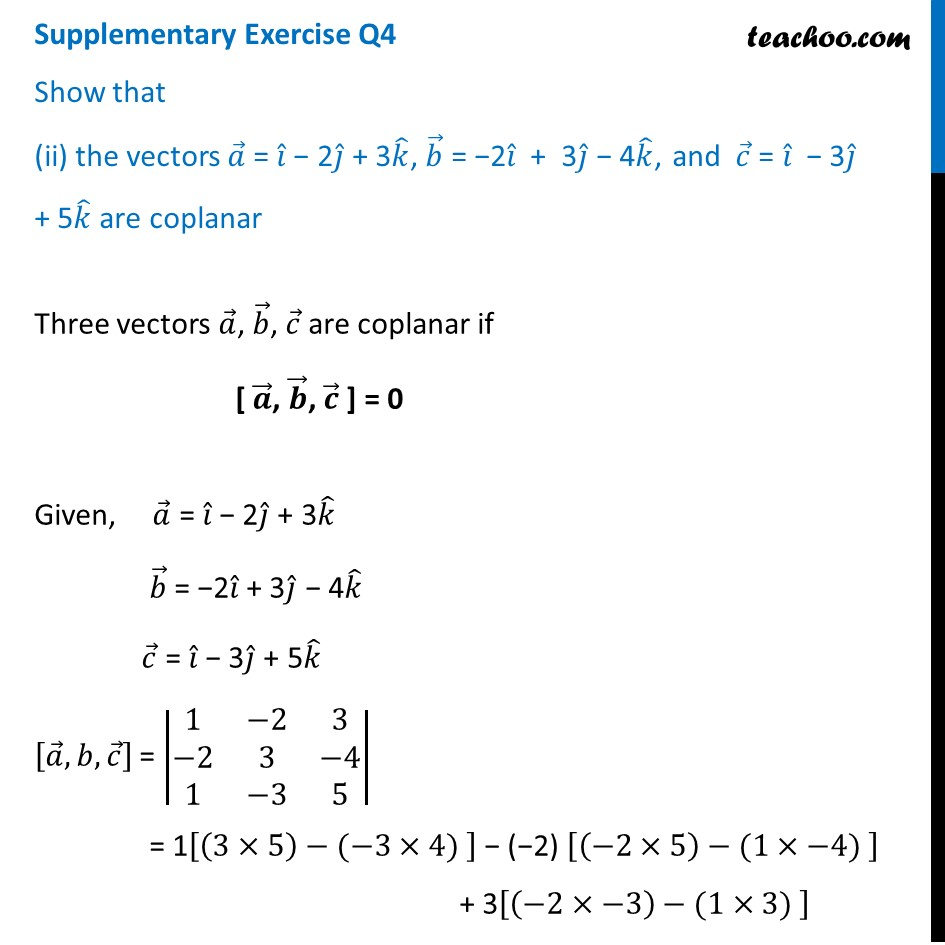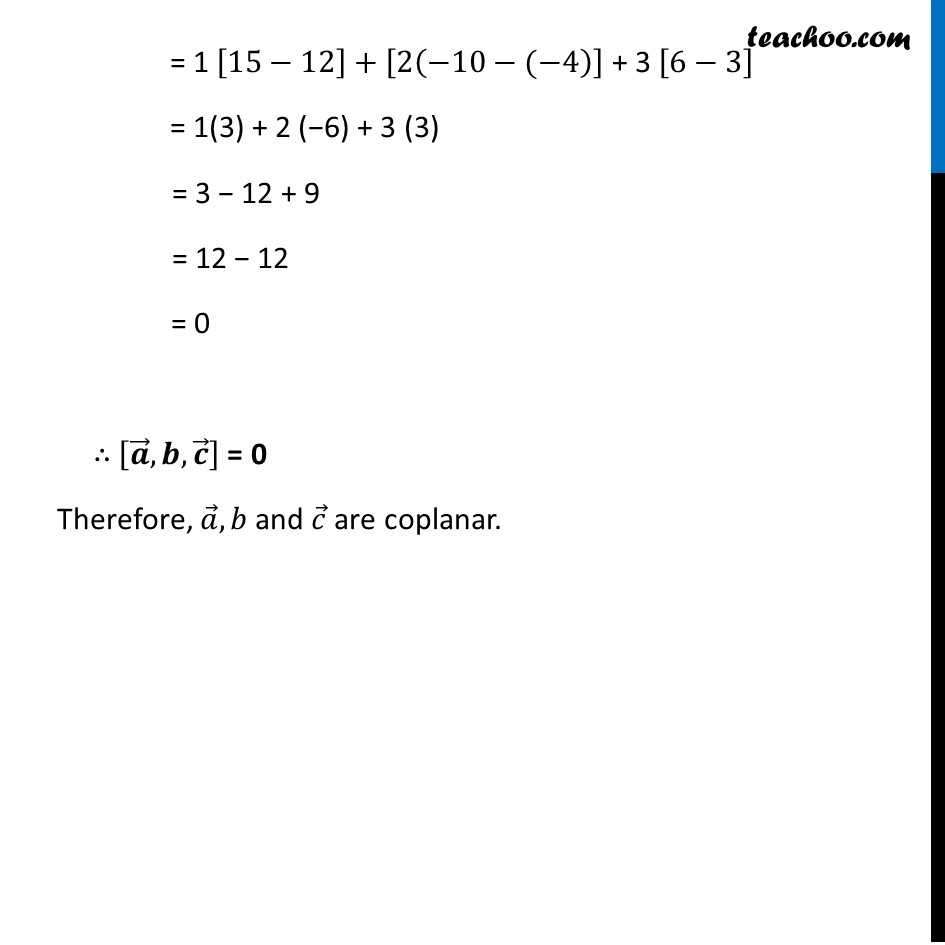Supplementary examples and questions from CBSE

Chapter 10 Class 12 Vector Algebra
Serial order wiseLearn in your speed, with individual attention - Teachoo Maths 1-on-1 Class

### Transcript

Supplementary Exercise Q4 Show that (i) the vectors 𝑎 ⃗ = 2𝑖 ̂ − 𝑗 ̂ + 𝑘 ̂, 𝑏 ⃗ = 𝑖 ̂ + 2𝑗 ̂ − 3𝑘 ̂, and 𝑐 ⃗ = 3𝑖 ̂ − 4𝑗 ̂ + 5𝑘 ̂ are coplanar. Three vectors 𝑎 ⃗, 𝑏 ⃗, 𝑐 ⃗ are coplanar if [ 𝒂 ⃗, 𝒃 ⃗, 𝒄 ⃗ ] = 0 Given, 𝑎 ⃗ = 2𝑖 ̂ − 𝑗 ̂ + 𝑘 ̂ 𝑏 ⃗ = 𝑖 ̂ + 2𝑗 ̂ − 3𝑘 ̂ 𝑐 ⃗ = 3𝑖 ̂ − 4𝑗 ̂ + 5𝑘 ̂ [𝑎 ⃗,𝑏,𝑐 ⃗ ] = |■8(2&−1&1@1&2&−3@3&−4&5)| = 2[(2×5)−(−4×−3)] − (−1) [(1×5)−(3×−3) ] + 1[(1×−4)−(3×2) ] = 2 [10−12]+[5+9] + [−4−6] = –4 + 14 – 10 = 0 ∴ [𝒂 ⃗,𝒃,𝒄 ⃗ ] = 0 Therefore, 𝑎 ⃗,𝑏 and 𝑐 ⃗ are coplanar. Supplementary Exercise Q4 Show that (ii) the vectors 𝑎 ⃗ = 𝑖 ̂ − 2𝑗 ̂ + 3𝑘 ̂, 𝑏 ⃗ = −2𝑖 ̂ + 3𝑗 ̂ − 4𝑘 ̂, and 𝑐 ⃗ = 𝑖 ̂ − 3𝑗 ̂ + 5𝑘 ̂ are coplanar Three vectors 𝑎 ⃗, 𝑏 ⃗, 𝑐 ⃗ are coplanar if [ 𝒂 ⃗, 𝒃 ⃗, 𝒄 ⃗ ] = 0 Given, 𝑎 ⃗ = 𝑖 ̂ − 2𝑗 ̂ + 3𝑘 ̂ 𝑏 ⃗ = −2𝑖 ̂ + 3𝑗 ̂ − 4𝑘 ̂ 𝑐 ⃗ = 𝑖 ̂ − 3𝑗 ̂ + 5𝑘 ̂ [𝑎 ⃗,𝑏,𝑐 ⃗ ] = |■8(1&−2&3@−2&3&−4@1&−3&5)| = 1[(3×5)−(−3×4) ] − (−2) [(−2×5)−(1×−4) ] + 3[(−2×−3)−(1×3) ] = 1 [15−12]+[2(−10−(−4)] + 3 [6−3] = 1(3) + 2 (−6) + 3 (3) = 3 − 12 + 9 = 12 − 12 = 0 ∴ [𝒂 ⃗,𝒃,𝒄 ⃗ ] = 0 Therefore, 𝑎 ⃗,𝑏 and 𝑐 ⃗ are coplanar.